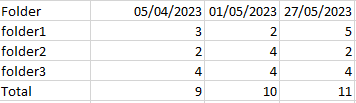cancel
Showing results for
Did you mean:Regular Visitor

## Calculate the increment of counted rows between dates

Hello,

I'm extracting the list of files in some folders every several days but not on a fix frequency. I need to calculate the increment of number of files between two extraction dates.

This is the look of the extraction table:I have a table visual with the total count per each folder per date:But I need to calculate the increment of files per folder between dates:Thank you very much in advance

1 ACCEPTED SOLUTIONSuper User

You could create a measure like

``````Increment =
VAR CurrentDate =
MAX ( 'Table'[Extract date] )
VAR PrevDate =
CALCULATE ( MAX ( 'Table'[Extract date] ), 'Table'[Extract date] < CurrentDate )
VAR CurrentNum =
COUNTROWS ( 'Table' )
VAR PrevNum =
CALCULATE ( COUNTROWS ( 'Table' ), 'Table'[Extract date] = PrevDate )
RETURN
CurrentNum - PrevNum
``````
6 REPLIES 6Super User

You could create a measure like

``````Increment =
VAR CurrentDate =
MAX ( 'Table'[Extract date] )
VAR PrevDate =
CALCULATE ( MAX ( 'Table'[Extract date] ), 'Table'[Extract date] < CurrentDate )
VAR CurrentNum =
COUNTROWS ( 'Table' )
VAR PrevNum =
CALCULATE ( COUNTROWS ( 'Table' ), 'Table'[Extract date] = PrevDate )
RETURN
CurrentNum - PrevNum
``````Regular Visitor

Hello Johnt75,

It works correctly, thank you very much. It just needs one small adjustment. If one folder that had files in the past has been deleted, the increment doesn't calculate the difference correctly, it has blank value. This is the result when creating a matrix with the increment:The folder "00-Executive Assistant" was deleted, so the countrows doesn't give any value for this folder and the increment is not calculated, it should indicate -10. Same happens in the first row of the table, one file that was in the root folder has been deleted but this is not indicated in the increment.

How can the formula be modified to consider removed folders?Super User

You could add "+ 0" to the CurrentRows variable. That would force it to return 0 instead of blank and should work out the difference correctly.Regular Visitor

I've tried to add "+0" in all the countrows and the final substraction but the result is exactly the same, I get blanks instead of the actual decrease of file numbers:

`Increment =VAR CurrentDate =`
`    MAX ( 'Listado'[Fecha extracto] )VAR PrevDate =    CALCULATE ( MAX ( 'Listado'[Fecha extracto] ), 'Listado'[Fecha extracto] < CurrentDate )VAR CurrentNum =      COUNTROWS ( 'Listado' ) + 0VAR PrevNum =      CALCULATE ( COUNTROWS ( 'Listado' ) + 0, 'Listado'[Fecha extracto] = PrevDate )RETURN    CurrentNum - PrevNum + 0`Super User

I think this is a problem caused by auto exist because everything is in one table. Try creating a table of all the distinct filenames, e.g.

``Filename dimension = DISTINCT( 'Listado'[Filename])``

link that to your main table in a one-to-many relationship and then use that new column in your visuals.Regular Visitor

I tried this solution but the visuals don't work, now they always give the total amount of files in the new table not considering the extract date.Announcements#### Exclusive opportunity for Women!

Join us for a free, hands-on Microsoft workshop led by women trainers for women where you will learn how to build a Dashboard in a Day!#### Power Platform Conference-Power BI and Fabric Sessions

Join us Oct 1 - 6 in Las Vegas for the Microsoft Power Platform Conference.Top Solution Authors
Top Kudoed Authors
Users online (2,923)